Anzeige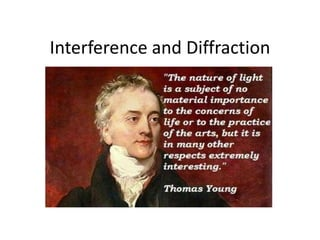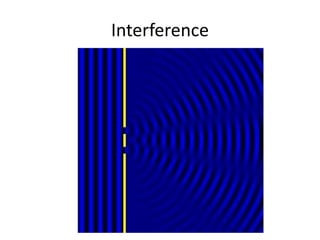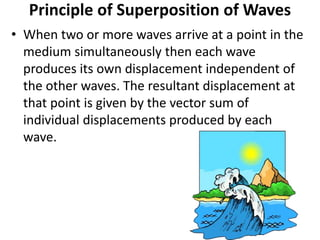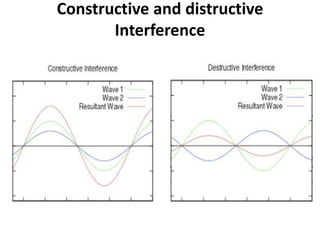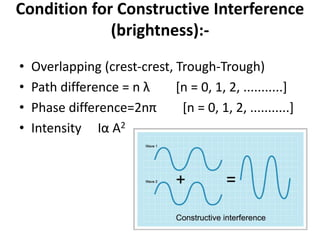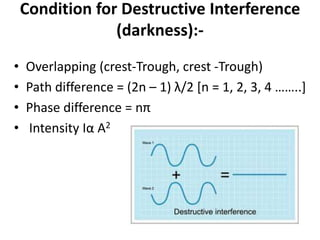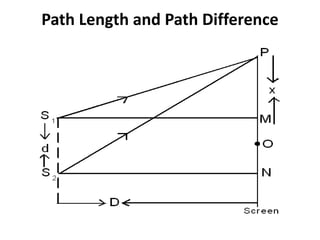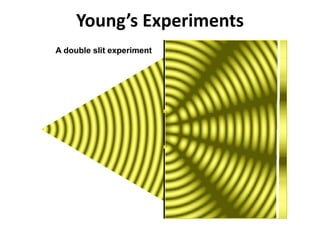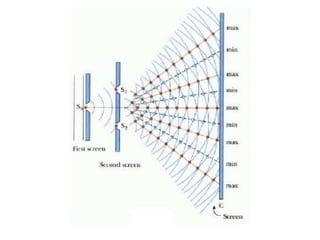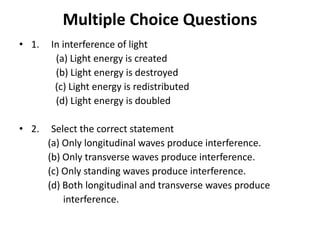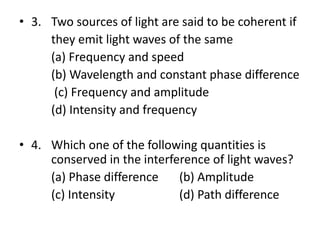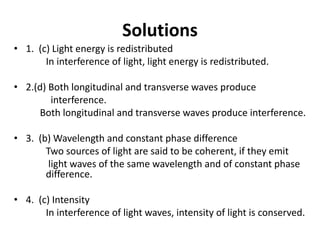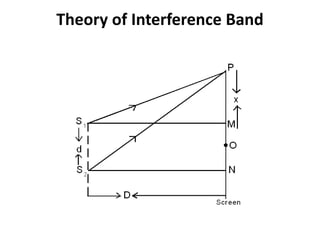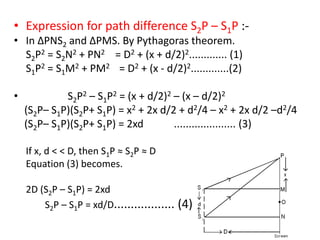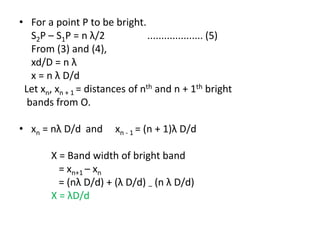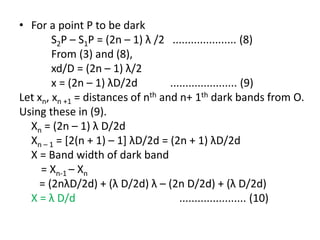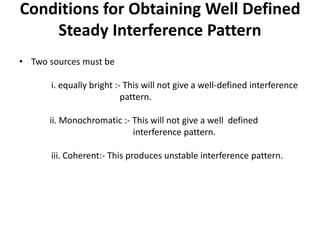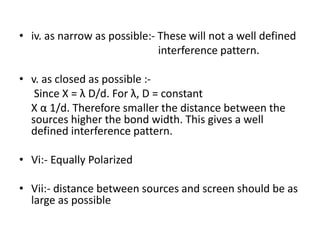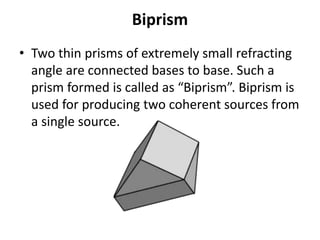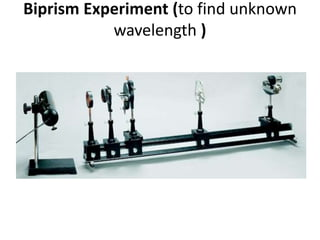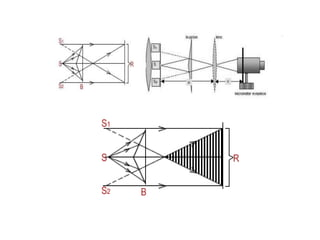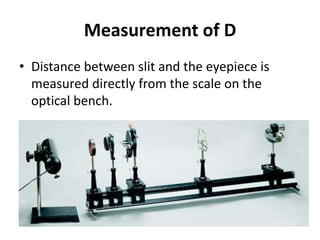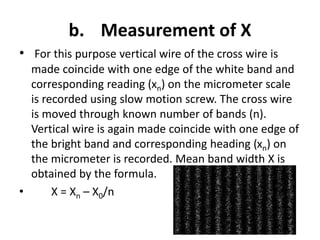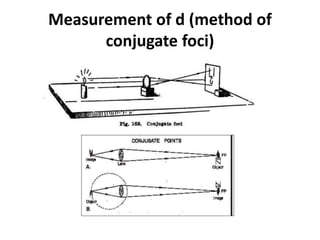1 von 24
Anzeige

### Summarized notes on Interference and Diffraction for JEE Main

1. Interference and Diffraction
2. Interference
3. Principle of Superposition of Waves • When two or more waves arrive at a point in the medium simultaneously then each wave produces its own displacement independent of the other waves. The resultant displacement at that point is given by the vector sum of individual displacements produced by each wave.
4. Constructive and distructive Interference
5. Condition for Constructive Interference (brightness):- • Overlapping (crest-crest, Trough-Trough) • Path difference = n λ [n = 0, 1, 2, ...........] • Phase difference=2nπ [n = 0, 1, 2, ...........] • Intensity Iα A2
6. Condition for Destructive Interference (darkness):- • Overlapping (crest-Trough, crest -Trough) • Path difference = (2n – 1) λ/2 [n = 1, 2, 3, 4 ……..] • Phase difference = nπ • Intensity Iα A2
7. Path Length and Path Difference
8. Young’s Experiments
9. Multiple Choice Questions • 1. In interference of light (a) Light energy is created (b) Light energy is destroyed (c) Light energy is redistributed (d) Light energy is doubled • 2. Select the correct statement (a) Only longitudinal waves produce interference. (b) Only transverse waves produce interference. (c) Only standing waves produce interference. (d) Both longitudinal and transverse waves produce interference.
10. • 3. Two sources of light are said to be coherent if they emit light waves of the same (a) Frequency and speed (b) Wavelength and constant phase difference (c) Frequency and amplitude (d) Intensity and frequency • 4. Which one of the following quantities is conserved in the interference of light waves? (a) Phase difference (b) Amplitude (c) Intensity (d) Path difference
11. Solutions • 1. (c) Light energy is redistributed In interference of light, light energy is redistributed. • 2.(d) Both longitudinal and transverse waves produce interference. Both longitudinal and transverse waves produce interference. • 3. (b) Wavelength and constant phase difference Two sources of light are said to be coherent, if they emit light waves of the same wavelength and of constant phase difference. • 4. (c) Intensity In interference of light waves, intensity of light is conserved.
12. Theory of Interference Band
13. • Expression for path difference S2P – S1P :- • In ∆PNS2 and ∆PMS. By Pythagoras theorem. S2P2 = S2N2 + PN2 = D2 + (x + d/2)2............. (1) S1P2 = S1M2 + PM2 = D2 + (x - d/2)2.............(2) • S2P2 – S1P2 = (x + d/2)2 – (x – d/2)2 (S2P– S1P)(S2P+ S1P) = x2 + 2x d/2 + d2/4 – x2 + 2x d/2 –d2/4 (S2P– S1P)(S2P+ S1P) = 2xd ..................... (3) If x, d < < D, then S1P ≈ S2P ≈ D Equation (3) becomes. 2D (S2P – S1P) = 2xd S2P – S1P = xd/D.................. (4)
14. • For a point P to be bright. S2P – S1P = n λ/2 .................... (5) From (3) and (4), xd/D = n λ x = n λ D/d Let xn, xn + 1 = distances of nth and n + 1th bright bands from O. • xn = nλ D/d and xn - 1 = (n + 1)λ D/d X = Band width of bright band = xn+1 – xn = (nλ D/d) + (λ D/d) – (n λ D/d) X = λD/d
15. • For a point P to be dark S2P – S1P = (2n – 1) λ /2 ..................... (8) From (3) and (8), xd/D = (2n – 1) λ/2 x = (2n – 1) λD/2d ...................... (9) Let xn, xn +1 = distances of nth and n+ 1th dark bands from O. Using these in (9). Xn = (2n – 1) λ D/2d Xn – 1 = [2(n + 1) – 1] λD/2d = (2n + 1) λD/2d X = Band width of dark band = Xn-1 – Xn = (2nλD/2d) + (λ D/2d) λ – (2n D/2d) + (λ D/2d) X = λ D/d ...................... (10)
16. Conditions for Obtaining Well Defined Steady Interference Pattern • Two sources must be i. equally bright :- This will not give a well-defined interference pattern. ii. Monochromatic :- This will not give a well defined interference pattern. iii. Coherent:- This produces unstable interference pattern.
17. • iv. as narrow as possible:- These will not a well defined interference pattern. • v. as closed as possible :- Since X = λ D/d. For λ, D = constant X α 1/d. Therefore smaller the distance between the sources higher the bond width. This gives a well defined interference pattern. • Vi:- Equally Polarized • Vii:- distance between sources and screen should be as large as possible
18. Biprism • Two thin prisms of extremely small refracting angle are connected bases to base. Such a prism formed is called as “Biprism”. Biprism is used for producing two coherent sources from a single source.
19. Biprism Experiment (to find unknown wavelength )
20. Measurement of D • Distance between slit and the eyepiece is measured directly from the scale on the optical bench.
21. b. Measurement of X • For this purpose vertical wire of the cross wire is made coincide with one edge of the white band and corresponding reading (xn) on the micrometer scale is recorded using slow motion screw. The cross wire is moved through known number of bands (n). Vertical wire is again made coincide with one edge of the bright band and corresponding heading (xn) on the micrometer is recorded. Mean band width X is obtained by the formula. • X = Xn – X0/n
22. Measurement of d (method of conjugate foci)
Anzeige